Tutorial math and reading software for elementary and secondary arithmetic, basic math, algebra, geometry, precalculus plus GED, ABE, and CLEP preparation for elementary school, high school, college, adult education, and homeschool students.

# Algebra help comes in a bundle price forthe first semester set of algebra software(Chapters 1-4)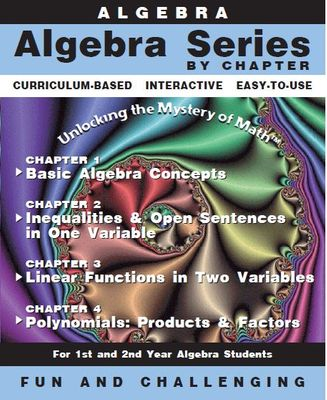Algebra Software
1st Semester Bundle (Ch 1 - 4)
Item# Alg-Ch1-4
Regular price: \$159.00
Limited Time Offer: \$79.50
Media type::

## Product Description

Each basic algebra chapter is organized as a traditional algebra 1 textbook. Learn algebra chapter by chapter.

Chapter 1: "Basic Algebra 1 Concepts" - Start with the basic algebra 1 concepts of real numbers, expressions, order of operation, algebraic properties, horizontal and vertical lines, sums & differences, products & quotients, solving equations, words to symbols and a comprehensive word problem solving section which includes the anatomy of a word problem, number problems, mixture problems, coin problems, finance problems, motion problems, and geometry problems. Learn algebra as each topic is introduced and explained with sample algebra problems followed by practice sets of algebra problems with the algebra solutions offered to the student step-by-step. This algebra software is your personal private algebra tutor.

Chapter 2: "Inequalities & Open Sentences in One Variable" - Basic algebra students and continuing algebra 2 students will learn algebra by working through inequalities and open sentences by solving inqualities, solving combined inequalities, absolute value inequalities, graphic solutions to inequalities and an "extras for experts" section on symbolic logic. Each topic is introduced and explained with sample algebra problems followed by practice sets of algebra problems with the algebra solutions offered to the student step-by-step. This algebra software is your personal private algebra tutor.

Chapter 3: "Linear Functions in Two Variables" - this math software provides algebra help and practice with linear equations, open sentences, graphing, comprehensive study of slope, standard form of a linear equation, horizontal and vertical lines and their graphs, forms of linear equations such as point-slope, slope-intercept, parallel & perpendicular slopes and their linear equations, writing the equation from the information given, systems of linear equations in two variables, linear inequalities, linear functions and relations. Each learning algebra topic is introduced and explained with sample algebra problems followed by practice sets of algebra problems with the algebra solutions offered to the student step-by-step. This algebra software is your personal private algebra tutor. Algebra 1 students will learn this material for the first time and algebra 2 students will review this very important material which is necessary for more advanced algebra studies. Math help is always available. MathMedia Educational Software will be your virtual math tutor.

Chapter 4: "Polynomials: Products & Factors" - Factoring polynomials is one of the most challenging topics both basic algebra and advanced algebra 2 students face. The in-depth math software chapter on factoring will provide the algebra help students require. Begin with an introduction to polynomials with algebra help and practice in the basic operations on polynomials, move on to exponents (definitions, rules, multiplication, division, power of a power, and even an exponent "challenge" section; continue with the multiplication of polynomials and prime factoring. Factoring polynomials provides the help and practice required to master common monomial factoring, common binomial factoring, the difference of two perfect squares, factoring cubes, factoring by grouping, factoring quadratics; conclude with solving polynomial equations and polynomial inequalities. Each topic is introduced and explained with sample algebra problems followed by practice sets of algebra problems with the algebra solutions offered to the student step-by-step. This algebra software is your personal private algebra tutor.

Each chapter offers the students comprehensive details for learning algebra with algebra help and algebra practice. Each math software chapter contains a separate algebra word problem solving section. Students with holes in their math education will receive the appropriate math help necessary for success.
Assessments
Every section of every program has a corresponding quiz to test the student's achievement. Scores are calculated and automatically sent to the included database. Use the quizzes for placement or accountability. Once the students have proven proficiency, they will take the Practice Test which contains questions from all the learning sections in the chapter and includes HINTs and solutions. After the Practice Test, students will take the concluding Chapter Test which will be scored and recorded.
Learning algebra - These four chapters represent the first half of a typical first year algebra course. Use this algebra software as a complete curriculum or within the curriculum or for after school enrichment or remediation. Students enjoy the interactivity of knowing immediately how to do a problem. MathMedia Educational Software will be your virtual math tutor. Algebra 2 students will use this software to review and prepare for the first semester of Algebra 2. (Functionality includes automatic scoring and recording of assessments as well as "bookmarking" for the student to return to the last screen they worked on in their previous session.)

Each chapter is available for \$49 or purchase this bundle set of 4 chapters for \$159.## Accessories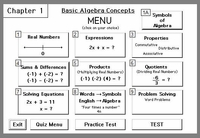Chapter 1 MENU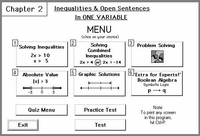Chapter 2 MENU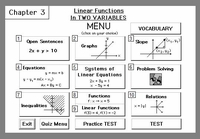Chapter 3 MENU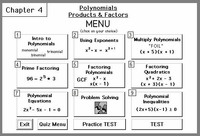Chapter 4 MENUChapter 1:Learning Basic Algebra ConceptsRegular price: \$49.00Limited Time Offer: \$24.50Chapter 2:Inequalities & Open Sentences in One VariableRegular price: \$49.00Limited Time Offer: \$24.50Chapter 3:Linear Equations in TWO VariablesRegular price: \$49.00Limited Time Offer: \$24.50Chapter 4:Polynomial Products & FactorsRegular price: \$49.00Limited Time Offer: \$24.50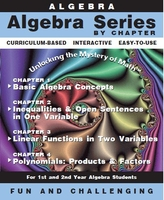Algebra Software1st Semester Bundle (Ch 1 - 4)Regular price: \$159.00Limited Time Offer: \$79.50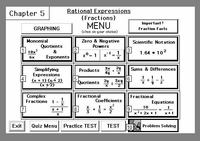Chapter 5:Rational Expressions(Algebraic Fractions)Regular price: \$49.00Limited Time Offer: \$24.50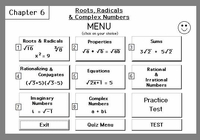Chapter 6:Square Roots, nth Roots, Radicals & Complex NumbersRegular price: \$49.00Limited Time Offer: \$24.50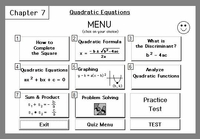Chapter 7:Quadratic EquationsRegular price: \$49.00Limited Time Offer: \$24.50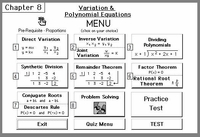Chapter 8:Variation and Advanced EquationsRegular price: \$49.00Limited Time Offer: \$24.50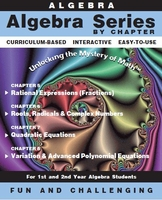Algebra Software By Chapter2nd Semester Bundle (Ch 5 - 8)Regular price: \$159.00Limited Time Offer: \$79.50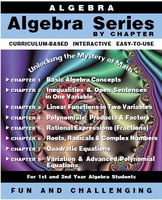Algebra Series By Chapter(Ch 1 - 8)Regular price: \$299.00Limited Time Offer: \$149.50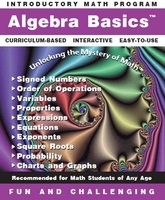Pre-Requisite for the Algebra Series - "Algebra Basics" (Pre-Algebra)Regular price: \$49.00Limited Time Offer: \$24.50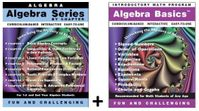Algebra By Chapter (8 Chapters) + Algebra BasicsRegular price: \$309.00Limited Time Offer: \$154.50Bundle #2High School Math Bundle(24 Programs + Algebra Readiness Test)Regular price: \$699.00Limited Time Offer: \$349.50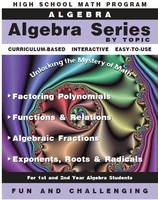Algebra by Topic(Set of Four Programs)Regular price: \$159.00Limited Time Offer: \$79.50